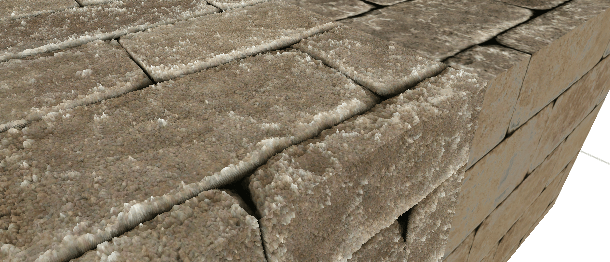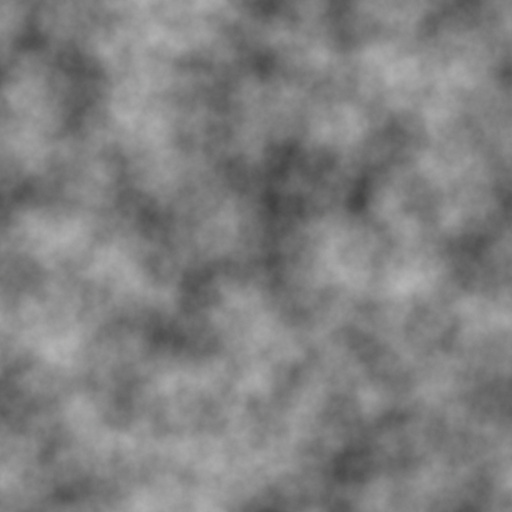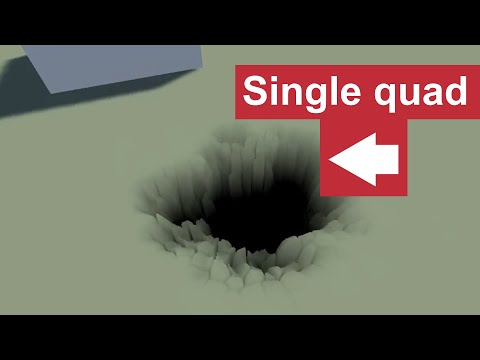## 3. Parallax mapping

When making a game it's really important to use as few triangles as possible. But what are you going to do if you for example have a brick wall and want to make those bricks more realistic and can't add more triangles? One solution is to add a normal map, but the result is still kinda flat. Another solution is to use parallax mapping. This is a comparison between bricks with parallax mapping and bricks without parallax mapping:Parallax mapping is a shader technique where you need to be able to write shaders on your own because you can't attach a parallax mapping shader made for bricks to simulate a landscape because you have to really tweak each shader to achive the best result. But the basic idea is the same. I'm not going to repeat what's already written, so if you want to read long articles about parallax mapping you can for example read this one: Learn OpenGL - Parallax Mapping. But I am going to tell you the basic idea:

• Like in the previous tutorials we are going to fire rays to simulate geometry where there is none.
• We are going to fire the rays not in global space, not in object space, but in tangent space. If you haven't heard about tangent space, you can say that two of the axis in tangent space are aligned with the u and v coordinates and the third axis is the normal to the surface.
• In the previous tutorials we used simple ray/geometry intersections to find where the ray has hit something. We can't do that here because the geometry is described with a grayscale texture. So we have to fire a ray with constant step size and each step we have to check if the ray is below the geometry.
• When the ray is below the geometry, then we need to find the intersection point by using a weighted average of the last point on the ray, which was above the surface. This will most likely not be the exact answer, so you might have to change the step size to get a more accurate answer.

If you are lost, maybe this image might explain it better:What we are going to do here is to simulate a landscape. If you are making a game where you are looking out of an aircraft window, then it's unnecessary to build the exact ground geometry with meshes, so you might as well just use a quad and parallax mapping. What you need is just a qaud, a noise texture, a mountain texture, and a grass texture. Google the mountain and grass textures, but you can use this seamless noise texture:```//Parallax occlusion mapping shader that creates a landscape in a quad
{
Properties
{
_NoiseTex("Noise texture", 2D) = "white" {}
_GrassTex("Grass (RGB)", 2D) = "white" {}
_StoneTex("Stone (RGB)", 2D) = "white" {}
_WaterColor("Water (RGB)", Color) = (1,1,1,1)
_Height("Height", Range(0.0001,5)) = 1.0
}

{
Tags { "RenderType"="Opaque" }
LOD 200

CGPROGRAM

#pragma surface surf Lambert vertex:vert
#pragma target 3.0

//Input
sampler2D _NoiseTex;
sampler2D _GrassTex;
sampler2D _StoneTex;
float4 _WaterColor;
float _Height;

struct Input
{
//What Unity can give you
float2 uv_NoiseTex;

//What you have to calculate yourself
float3 tangentViewDir;
};

void vert(inout appdata_full i, out Input o)
{
UNITY_INITIALIZE_OUTPUT(Input, o);

//Transform the view direction from world space to tangent space
float3 worldVertexPos = mul(unity_ObjectToWorld, i.vertex).xyz;
float3 worldViewDir = worldVertexPos - _WorldSpaceCameraPos;

//To convert from world space to tangent space we need the following
float3 worldNormal = UnityObjectToWorldNormal(i.normal);
float3 worldTangent = UnityObjectToWorldDir(i.tangent.xyz);
float3 worldBitangent = cross(worldNormal, worldTangent) * i.tangent.w * unity_WorldTransformParams.w;

//Use dot products instead of building the matrix
o.tangentViewDir = float3(
dot(worldViewDir, worldTangent),
dot(worldViewDir, worldNormal),
dot(worldViewDir, worldBitangent)
);
}

//Animate the uv coordinates so the landscape is moving
float2 animateUV(float2 texturePos)
{
texturePos.x += _Time * 5;

return texturePos;
}

//Get the height from a uv position
float getHeight(float2 texturePos)
{
texturePos = animateUV(texturePos);

//Multiply with 0.2 to make the landscape flatter
float4 colorNoise = tex2Dlod(_NoiseTex, float4(texturePos * 0.2, 0, 0));

//Calculate the height at this uv coordinate
//Just use r because r = g = b  because color is grayscale
//(1-color.r) because black should be low
//-1 because the ray is going down so the ray's y-coordinate will be negative
float height = (1 - colorNoise.r) * -1 * _Height;

return height;
}

//Combine stone and grass depending on grayscale color
float4 getBlendTexture(float2 texturePos, float height)
{
texturePos = animateUV(texturePos);

//To make it look nice by making the texture a little bigger
float textureSize = 0.4;

float4 colorGrass = tex2Dlod(_GrassTex, float4(texturePos * textureSize, 0, 0));
float4 colorStone = tex2Dlod(_StoneTex, float4(texturePos * textureSize, 0, 0));

//Height is negative so convert it to positive, also invert it so mountains are high and not the grass
//Divide with _Height because this height is actual height and we need it in 0 -> 1 range
float colorGrayscale = 1 - (abs(height) / _Height);

//Combine grass and stone depending on height
float4 mixedColor = lerp(colorGrass, colorStone, colorGrayscale);

//Water
if (colorGrayscale < 0.4)
{
mixedColor = tex2Dlod(_NoiseTex, float4(texturePos * 0.2, 0, 0)) * _WaterColor;
}

return mixedColor;
}

//Get the texture position by interpolation between the position where we hit terrain and the position before
float2 getWeightedTexPos(float3 rayPos, float3 rayDir, float stepDistance)
{
//Move one step back to the position before we hit terrain
float3 oldPos = rayPos - stepDistance * rayDir;

float oldHeight = getHeight(oldPos.xz);

//Always positive
float oldDistToTerrain = abs(oldHeight - oldPos.y);

float currentHeight = getHeight(rayPos.xz);

//Always negative
float currentDistToTerrain = rayPos.y - currentHeight;

float weight = currentDistToTerrain / (currentDistToTerrain - oldDistToTerrain);

//Calculate a weighted texture coordinate
//If height is -2 and oldHeight is 2, then weightedTex is 0.5, which is good because we should use
//the exact middle between the coordinates
float2 weightedTexPos = oldPos.xz * weight + rayPos.xz * (1 - weight);

return weightedTexPos;
}

void surf (Input IN, inout SurfaceOutput o)
{
//Where is the ray starting? y is up and we always start at the surface
float3 rayPos = float3(IN.uv_NoiseTex.x, 0, IN.uv_NoiseTex.y);

//What's the direction of the ray?
float3 rayDir = normalize(IN.tangentViewDir);

//Find where the ray is intersecting with the terrain with a raymarch algorithm
int STEPS = 300;
float stepDistance = 0.01;

//The default color used if the ray doesnt hit anything
float4 finalColor = 1;

for (int i = 0; i < STEPS; i++)
{
//Get the current height at this uv coordinate
float height = getHeight(rayPos.xz);

//If the ray is below the surface
if (rayPos.y < height)
{
//Get the texture position by interpolation between the position where we hit terrain and the position before
float2 weightedTex = getWeightedTexPos(rayPos, rayDir, stepDistance);

float height = getHeight(weightedTex);

finalColor = getBlendTexture(weightedTex, height);

//We have hit the terrain so we dont need to loop anymore
break;
}

//Move along the ray
rayPos += stepDistance * rayDir;
}

//Output
o.Albedo = finalColor.rgb;
}
ENDCG
}
FallBack "Diffuse"
}
```